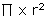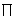# Why Is It Important that Someone on This Planet Understands the Geometry of a Rectangle?

Article Summary: What do you know about the geometry of a rectangle? To calculate the perimeter of a rectangle, the distance around the outside, you simply add out the length of the four sides. Rectangles and squares are found everywhere around you. Make a list of all the things around you at this moment that are rectangular or square.

Do you remember the definition of a rectangle? A rectangle has four sides and opposite sides are parallel plus the each of the four corners is 90 degrees or perpendicular. That's a complicated definition for a shape that you see all the time. Why, the computer monitor screen that you are looking at right now is probably an example of a rectangle. A square is a specialized rectangle where all the sides are equal.

What do you know about the geometry of a rectangle? To calculate the perimeter of a rectangle, the distance around the outside, you simply add out the length of the four sides. An even easier formula is

Perimeter of a rectangle = 2 x length + 2 x width

The area of a rectangle is also easy to calculate.

Area of a rectangle = length x width

Rectangles and squares are found everywhere around you. Make a list of all the things around you at this moment that are rectangular or square. A possible list includes paper, Post-it notes, calendar, books, windows, walls, ceiling, floor, the top of the desk, sides of the desk, desk drawers, waste basket and calculator. What else do you see that is rectangular or square?

If you have a tile floor, you may see square tiles or rectangular tiles. If you have a wooden floor, each board is a long and narrow rectangle but it's still a rectangle. Maybe you have carpet that covers the entire floor. The room you are in is most likely a rectangle or square. Think about how easy it is to figure out how much flooring you need to buy.

Room is 10 feet by 12 feet

Area to cover is 10 feet x 12 feet = 120 square feet

If you want to buy floor tile and each tile is a 12 inch square, how many tiles do you need for the room? Each tile is 1 square foot, so you need 120 tiles.

Wooden flooring is a little more complicated because boards can be 3 inches wide and 8 feet long. Each board would cover 1/4 foot x 8 feet or 2 square feet. So you would need 60 boards.

Carpet can be just as easy or a little harder depending on the size of the room. Carpet comes in rolls that are either 12 feet or 14 feet wide. This time it's easy - just measure 10 feet of carpet off of the roll!

Rectangles and squares are just easy to work with. Imagine if your room was circular and you wanted to put in new flooring. Remember that the area of a circle is calculated as

Area of a circle =is the number 3.14 and r2 is the radius times itself. But the radius is measured from the center point of the circle to the outside of the circle or the radius is half the diameter, the distance across the circle again at the center point.

Do you have a headache already from trying to put new flooring into a circular room? The geometry of a rectangle or square is so easy to work with that land measurements like house lots, building measurements and lots of other useful everyday objects are rectangular or square.

Another reason that so many buildings are rectangular is that straight walls and floors have built-in strength. We say that they are structurally sound because it's easy to put in studs, upright boards inside the walls, to support the structure. Your house or school probably has studs every two feet inside the walls.

Do you now have some idea why it is important that someone on this planet understands the geometry of a rectangle?